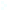Election CitationResearch Interests Name Demmel, James W. Location University of California, Berkeley Primary Field Applied Mathematical Sciences Secondary Field Computer and Information Sciences Demmel is at the center of numerical linear algebra, including theory, software (especially for advanced computing), and applications. Beginning with defining a geometric interpretation of ill-posed problems, Demmel?s accomplishments range from creation of algorithms with rigorous mathematical foundations to development of widely available software (LAPACK, ScaLAPACK, and SuperLU). James W. Demmel's research interests are in high performance computing and numerical algorithms, in particular for linear algebra, where he has helped develop the widely used software libraries LAPACK, ScaLAPACK and SuperLU. His primary research motivation is that the most expensive operations on current and future computers (measured in time or energy) are not arithmetic operations, but operations that just move data, either between levels of a memory hierarchy, or between parallel processors connected by a network. This means that the cost of many algorithms is dominated by moving data, not arithmetic. He thus seeks to (1) prove lower bounds on data movement necessary to solve common problems in computational science and engineering (such as solving linear systems of equations, least squares problems, eigenvalue problems, and computing the singular value decomposition) and (2) design new algorithms that attain these lower bounds. These new algorithms can be faster than previous ones by large factors. Additionally, he works on identifying which structured matrix problems have the property that efficient algorithms exist that guarantee accurate solutions, i.e. some leading digits of the answer are guaranteed correct, no matter how ill-conditioned the solution is, without needing arbitrary precision arithmetic.